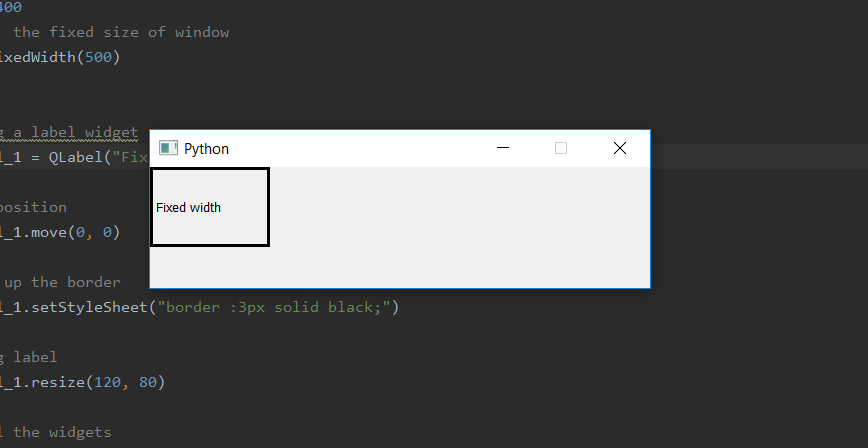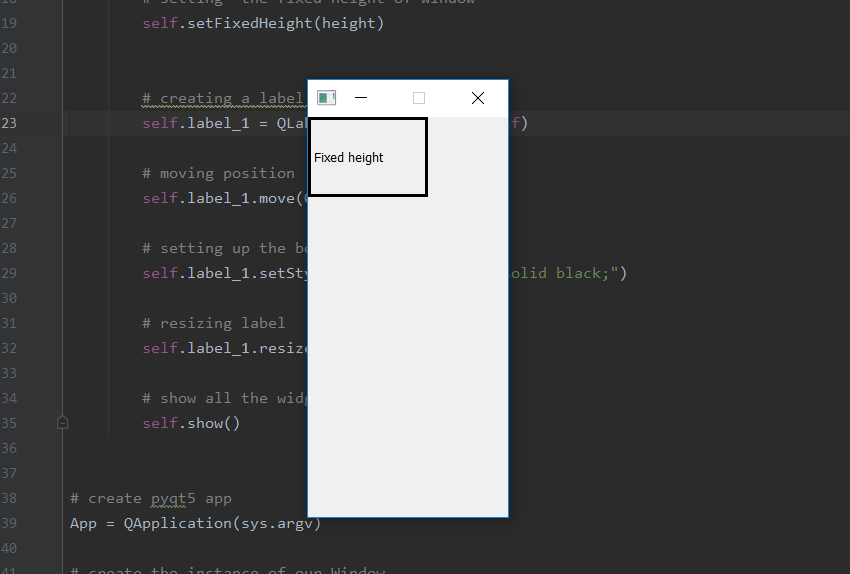# PyQt5 – Set fix window size for height or width

When we create a window, by default the window size is resizable although, we can use `setFixedSize()` method to set the fixed size of the window but if we want to set only fix length of height or width only we cant use this method. We want to set one length fixed and other be variable in order to do so we have to use `setFixedWidth()` method to set fix length of width and `setFizedHeight()` method to set fix length of height.

Syntax :

```self.setFixedWidth(width)
self.setFixedHeight(height)
```

Argument : Both take integer as argument.

Action performed:
`setFixedWidth()` sets the constant width.
`setFixedWidth()` sets the constant height.

Code for fixed width –

 `# importing the required libraries ` ` `  `from` `PyQt5.QtCore ``import` `*`  `from` `PyQt5.QtGui ``import` `*`  `from` `PyQt5.QtWidgets ``import` `*`  `import` `sys ` ` `  ` `  `class` `Window(QMainWindow): ` `    ``def` `__init__(``self``): ` `        ``super``().__init__() ` ` `  ` `  `        ``# set the title ` `        ``self``.setWindowTitle(``"Python"``) ` ` `  `        ``width ``=` `500` `         `  `        ``# setting  the fixed width of window ` `        ``self``.setFixedWidth(width) ` ` `  ` `  `        ``# creating a label widget ` `        ``self``.label_1 ``=` `QLabel(``"Fixed width"``, ``self``) ` ` `  `        ``# moving position ` `        ``self``.label_1.move(``0``, ``0``) ` ` `  `        ``# setting up the border ` `        ``self``.label_1.setStyleSheet(``"border :3px solid black;"``) ` ` `  `        ``# resizing label ` `        ``self``.label_1.resize(``120``, ``80``) ` ` `  `        ``# show all the widgets ` `        ``self``.show() ` ` `  ` `  `# create pyqt5 app ` `App ``=` `QApplication(sys.argv) ` ` `  `# create the instance of our Window ` `window ``=` `Window() ` ` `  `# start the app ` `sys.exit(App.``exec``()) `

Output :Code for fixed height –

 `# importing the required libraries ` ` `  `from` `PyQt5.QtCore ``import` `*`  `from` `PyQt5.QtGui ``import` `*`  `from` `PyQt5.QtWidgets ``import` `*`  `import` `sys ` ` `  ` `  `class` `Window(QMainWindow): ` `    ``def` `__init__(``self``): ` `        ``super``().__init__() ` ` `  ` `  `        ``# set the title ` `        ``self``.setWindowTitle(``"Python"``) ` ` `  `        ``height ``=` `400` `        ``# setting  the fixed height of window ` `        ``self``.setFixedHeight(height) ` ` `  ` `  `        ``# creating a label widget ` `        ``self``.label_1 ``=` `QLabel(``"Fixed height"``, ``self``) ` ` `  `        ``# moving position ` `        ``self``.label_1.move(``0``, ``0``) ` ` `  `        ``# setting up the border ` `        ``self``.label_1.setStyleSheet(``"border :3px solid black;"``) ` ` `  `        ``# resizing label ` `        ``self``.label_1.resize(``120``, ``80``) ` ` `  `        ``# show all the widgets ` `        ``self``.show() ` ` `  ` `  `# create pyqt5 app ` `App ``=` `QApplication(sys.argv) ` ` `  `# create the instance of our Window ` `window ``=` `Window() ` ` `  `# start the app ` `sys.exit(App.``exec``()) `

Output :My Personal Notes arrow_drop_upCheck out this Author's contributed articles.

If you like GeeksforGeeks and would like to contribute, you can also write an article using contribute.geeksforgeeks.org or mail your article to contribute@geeksforgeeks.org. See your article appearing on the GeeksforGeeks main page and help other Geeks.

Please Improve this article if you find anything incorrect by clicking on the "Improve Article" button below.

Article Tags :

Be the First to upvote.

Please write to us at contribute@geeksforgeeks.org to report any issue with the above content.